## First-Order Homogeneous Equations

A function f( x,y) is said to be homogeneous of degree n if the equationholds for all x,y, and z (for which both sides are defined).

Example 1: The function f( x,y) = x 2 + y 2 is homogeneous of degree 2, since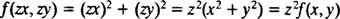Example 2: The functionis homogeneous of degree 4, since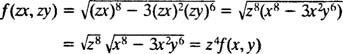Example 3: The function f( x,y) = 2 x + y is homogeneous of degree 1, sinceExample 4: The function f( x,y) = x 3y 2 is not homogeneous, since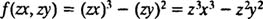which does not equal z n f( x,y) for any n.

Example 5: The function f( x,y) = x 3 sin ( y/x) is homogeneous of degree 3, sinceA first‐order differential equation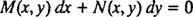is said to be homogeneous if M( x,y) and N( x,y) are both homogeneous functions of the same degree.

Example 6: The differential equation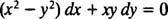is homogeneous because both M( x,y) = x 2y 2 and N( x,y) = xy are homogeneous functions of the same degree (namely, 2).

The method for solving homogeneous equations follows from this fact:

The substitution y = xu (and therefore dy = xdu + udx) transforms a homogeneous equation into a separable one.

Example 7: Solve the equation ( x 2y 2) dx + xy dy = 0.

This equation is homogeneous, as observed in Example 6. Thus to solve it, make the substitutions y = xu and dy = x dy + u dx: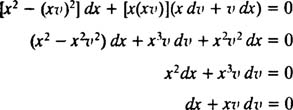This final equation is now separable (which was the intention). Proceeding with the solution,Therefore, the solution of the separable equation involving x and v can be written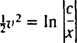To give the solution of the original differential equation (which involved the variables x and y), simply note that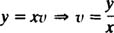Replacing v by y/ x in the preceding solution gives the final result:This is the general solution of the original differential equation.

Example 8: Solve the IVP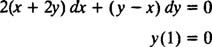Since the functions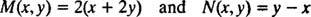are both homogeneous of degree 1, the differential equation is homogeneous. The substitutions y = xv and dy = x dv + v dx transform the equation intowhich simplifies as follows: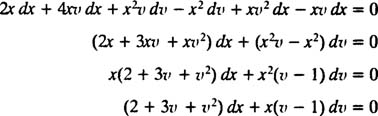The equation is now separable. Separating the variables and integrating gives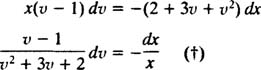The integral of the left‐hand side is evaluated after performing a partial fraction decomposition:Therefore,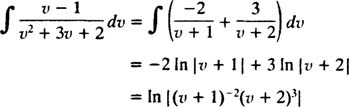The right‐hand side of (†) immediately integrates to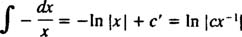Therefore, the solution to the separable differential equation (†) is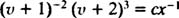Now, replacing v by y/ x gives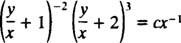as the general solution of the given differential equation. Applying the initial condition y(1) = 0 determines the value of the constant c: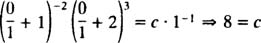Thus, the particular solution of the IVP iswhich can be simplified to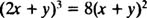as you can check.

Technical note: In the separation step (†), both sides were divided by ( v + 1)( v + 2), and v = –1 and v = –2 were lost as solutions. These need not be considered, however, because even though the equivalent functions y = – x and y = –2 x do indeed satisfy the given differential equation, they are inconsistent with the initial condition.

Back to Top
A18ACD436D5A3997E3DA2573E3FD792A